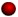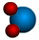Chemical Relationships

# Chemical Equations (previous version)

by Anthony Carpi, Ph.D.

An updated version of the Chemical Equations module is available. To view it, go here.

Chemical reactions happen all around us: when we light a match, start a car, eat dinner, or walk the dog. A chemical reaction is the process by which substances bond together (or break bonds) and, in doing so, either release or consume energy (see our Chemical Reactions module). A chemical equation is shorthand that scientists use to describe a chemical reaction. Let's take the reaction of hydrogen with oxygen to form water as an example. If we had a container of hydrogen gas and burned this in the presence of oxygen, the two gases would react together, releasing energy, to form water. To write the chemical equation for this reaction, we would place the substances reacting (the reactants) on the left side of an equation with an arrow pointing to the substances being formed on the right side of the equation (the products). Given this information, one might guess that the equation for this reaction is written:

 H + O → H2O

The plus sign on the left side of the equation means that hydrogen (H) and oxygen (O) are reacting. Unfortunately, there are two problems with this chemical equation. First, because atoms like to have full valence shells, single H or O atoms are rare. In nature, both hydrogen and oxygen are found as diatomic molecules, H2 and O2, respectively (in forming diatomic molecules the atoms share electrons and complete their valence shells). Hydrogen gas, therefore, consists of H2 molecules; oxygen gas consists of O2. Correcting our equation we get:

 H2 + O2 → H2O

But we still have one problem. As written, this equation tells us that one hydrogen molecule (with two H atoms) reacts with one oxygen molecule (two O atoms) to form one water molecule (with two H atoms and one O atom). In other words, we seem to have lost one O atom along the way!

## Balancing equations

To write a chemical equation correctly, the number of atoms on the left side of a chemical equation has to be precisely balanced with the atoms on the right side of the equation. How does this happen? In actuality, the O atom that we "lost" reacts with a second molecule of hydrogen to form a second molecule of water. During the reaction, the H-H and O-O bonds break and H-O bonds form in the water molecules, as seen in the simulation below.

The balanced equation is therefore written:

 2H2 + O2 → 2H2O

In writing chemical equations, the number in front of the molecule's symbol (called a coefficient) indicates the number of molecules participating in the reaction. If no coefficient appears in front of a molecule, we interpret this as meaning one.

In order to write a correct chemical equation, we must balance all of the atoms on the left side of the reaction with the atoms on the right side. Let's look at another example. If you use a gas stove to cook your dinner, chances are that your stove burns natural gas, which is primarily methane. Methane (CH4) is a molecule that contains four hydrogen atoms bonded to one carbon atom. When you light the stove, you are supplying the activation energy to start the reaction of methane with oxygen in the air. During this reaction, chemical bonds break and re-form and the products that are produced are carbon dioxide and water vapor (and, of course, light and heat that you see as the flame). The unbalanced chemical equation would be written:

 CH4 (methane) + O2 (oxygen) → CO2 (carbon dioxide) + H2O (water)

Look at the reaction atom by atom. On the left side of the equation we find one carbon atom, and one on the right.

 C H4 + O2 → C O2 + H2 O ↑ 1 carbon ↑ 1 carbon

Next we move to hydrogen: There are four hydrogen atoms on the left side of the equation, but only two on the right.

 C H4 + O2 → C O2 + H2 O ↑ 4 hydrogen ↑ 2 hydrogen

Therefore, we must balance the H atoms by adding the coefficient "2" in front of the water molecule (you can only change coefficients in a chemical equation, not subscripts). Adding this coefficient we get:

 C H4 + O2 → C O2 + 2H2 O ↑ 4 hydrogen ↑ 4 hydrogen

What this equation now says is that two molecules of water are produced for every one molecule of methane consumed.  Moving on to the oxygen atoms, we find two on the left side of the equation, but a total of four on the right side (two from the CO2 molecule and one from each of two water molecules H2O).

 C H4 + O2 → C O2 + 2H2 O ↑ 2 oxygen ↑ 4 oxygen

To balance the chemical equation we must add the coefficient "2" in front of the oxygen molecule on the left side of the equation, showing that two oxygen molecules are consumed for every one methane molecule that burns.

 C H4 + 2O2 → C O2 + 2H2 O ↑ 4 oxygen ↑ 4 oxygen

Comprehension Checkpoint

There is the same number of _____ on both sides of a balanced equation.

## Dalton's law of definite proportions

Dalton's law of definite proportions holds true for all chemical reactions (see our Early Ideas about Matter: From Democritus to Dalton module). In essence, this law states that a chemical reaction always proceeds according to the ratio defined by the balanced chemical equation. Thus, you can interpret the balanced methane equation above as reading, "one part methane reacts with two parts oxygen to produce one part carbon dioxide and two parts water." This ratio always remains the same. For example, if we start with two parts methane, then we will consume four parts O2 and generate two parts CO2 and four parts H2O. If we start with excess of any of the reactants (e.g., five parts oxygen when only one part methane is available), the excess reactant will not be consumed:

 C H4 + 5O2 → C O2 + 2H2 + 0 + 3O2 Excess reactants will not be consumed.

In the example seen above, 3O2 had to be added to the right side of the equation to balance it and show that the excess oxygen is not consumed during the reaction. In this example, methane is called the limiting reactant.

Although we have discussed balancing equations in terms of numbers of atoms and molecules, keep in mind that we never talk about a single atom (or molecule) when we use chemical equations. This is because single atoms (and molecules) are so tiny that they are difficult to isolate. Chemical equations are discussed in relation to the number of moles of reactants and products used or produced (see our The Mole module). Because the mole refers to a standard number of atoms (or molecules), the term can simply be substituted into chemical equations. Thus, the balanced methane equation above can also be interpreted as reading, "one mole of methane reacts with two moles of oxygen to produce one mole of carbon dioxide and two moles of water."

Comprehension Checkpoint

Dalton's law of definite proportions states that in chemical reactions,

## Conservation of matter

The law of conservation of matter states that matter is neither lost nor gained in traditional chemical reactions; it simply changes form. Thus, if we have a certain number of atoms of an element on the left side of an equation, we have to have the same number on the right side. This implies that mass is also conserved during a chemical reaction. The water reaction, for example:

 2H2 + O2 → 2H2O+→2 * 2.02g + 32.00g = 2 * 18.02g

The total mass of the reactants, 36.04g, is exactly equal to the total mass of the products, 36.04g (if you are confused about these molecular weights, you should review the The Mole lesson). This holds true for all balanced chemical equations.

### Summary

When scientists want to describe a chemical reaction in writing, they use precise chemical equations. This module, the third in a series on chemical reactions, explains chemical equations in a step-by-step process. The module shows how chemical equations are balanced in the context of the chemical changes that take place during a reaction. The Law of Conservation of Matter is introduced.

• ##### NGSS
• HS-C3.5, HS-C5.1, HS-PS1.B1, HS-PS1.B3

Anthony Carpi, Ph.D. “Chemical Equations (previous version)” Visionlearning Vol. CHE-1 (8), 2003.

Top